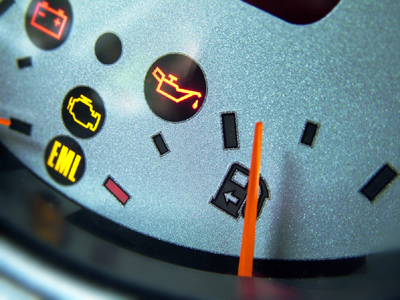For every 4 liters of gasoline a car can travel 80km. 5 liters of gasoline is required for a journey of 100km.

# Ratio 2

This Math quiz is called 'Ratio 2' and it has been written by teachers to help you if you are studying the subject at middle school. Playing educational quizzes is a fabulous way to learn if you are in the 6th, 7th or 8th grade - aged 11 to 14.

It costs only \$12.50 per month to play this quiz and over 3,500 others that help you with your school work. You can subscribe on the page at Join Us

Ratio is the relation between two numbers or quantities. It is useful for showing the number of times one value contains or is contained within the other. For instance in the ratio 1:3, if 1 is sand and 3 is water, you would need three times the amount of water in relation to sand. Ratios are excellent tools which help us to visualize how different numbers of things compare to one another. They are also very similar to fractions. The ratios 1:3 and 2:3 can also be represented by the fractions 13 and 23

1.
The proportion of brick houses in every 20 is 310. How many brick houses in a street of 100 houses?
6
10
30
50
310 of 20 = 6. So there are 6 brick houses in every 20. 100 is 5 times 20 so we multiply 6 by 5
2.
A cookie recipe needs 250g flour, 150g of sugar and 150g of butter to make 6 cookies. How much butter is needed to make 18 cookies?
150g
300g
400g
450g
18 is 3 x 6 so we multiply 150g by 3
3.
In every 12 tiles the proportion of blue tiles is 34. How many blue tiles in every 12?
3
6
8
9
34 of 12 is 9
4.
Jo mixes 400ml of orange juice to every 200ml of pineapple juice to make a fruit cocktail. How much orange juice will she need to make 900ml of fruit cocktail?
200ml
400ml
600ml
800ml
There is 400ml in every 600ml. 900ml = 1.5 x 600ml. So multiply 400ml by 1.5
5.
In every 10 tiles there are 2 red tiles. What is the proportion of red tiles?
13
14
15
12
210 and 2:10 are the same as 15 and 1:5
6.
For every 4 liters of gasoline a car can travel 80km. How much gasoline is required for a journey of 100km?
5 liters
8 liters
10 liters
12 liters
If 4 liters can travel 80km we divide by 4 to find 1 liter will travel 20km. So 5 liters will travel 100km
7.
There are 2 white cubes for every 1 blue cube. What proportion are blue cubes to white cubes?
13
14
15
12
12 is the same as 1:2
8.
In a grid of 10 squares 4 are white and the rest are blue. What is the ratio of white to blue?
1:2
2:4
4:6
5:6
10 - 4 = 6 so there are 6 blue squares for every 4 white squares
9.
If there is a 10:1 ratio between black squares and white squares which number represents white squares?
1
2
5
10
The 10 represents black and the 1 represents white because black was mentioned first and white second
10.
In a box of chocolates there are 4 milk chocolates to 3 dark chocolates. How many dark chocolates in a box of 49 chocolates?
7
21
28
30
There are 3 dark chocolates in every 7. There are seven 7s in 49 so we multiply 3 by 7
Author:  Amanda Swift